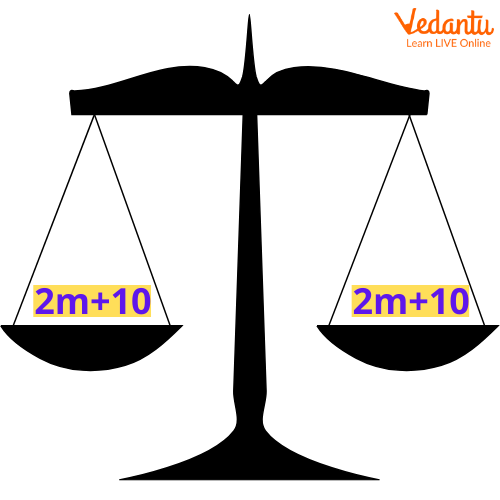Courses
Courses for Kids
Free study material
Free LIVE classes
More

# Property of EqualityLIVE
Join Vedantu’s FREE Mastercalss

## What is Equality in Maths?

In Mathematics, two things are equal if and only if they are exactly similar in every way i.e. they have the same Mathematical value and Mathematical properties. Mathematics uses the equality sign (=) to represent equality. For example, the statement A = B implies that A and B are equal.

## What is the Property of Equality?

Property of equality is the fact or truth that is applied to two or more quantities related by an equal sign. Let us learn different properties of equality with examples.The Addition property of equality states that when the same number is added from both sides of the equation, the equation still holds true. In other words, it states that if,

X = Y,

then X + Z = Y + Z

Example:

We know that, 4 + 3 = 7

Here, if we add 5 to both sides of the equation, the equation still holds true.

i.e. 4 + 3 + 5 = 7 + 5

12 = 12

LHS = RHS

## Subtraction Property of Equality

The subtraction property of equality states that when the same number is subtracted from both sides of the equation, the equation still holds. In other words it states that if,

X = Y, then X -  Z = Y -  Z

Example:

We know that, 9 - 3 = 6

Here, if we subtract 2 to both sides of the equation, the equation still holds

9 - 3 - 2 = 6 - 2

4  = 4

LHS = RHS

## Multiplication Property of Equality

The multiplication property of equality states that when both sides of an equation are multiplied by the same number, the two sides remain equal.

In other words, it states that if x, y, and z are real numbers such that x = y, then

a x c  = b x c .

Example, If a = 10, b = 10, and c = 12, then

a x c = b x

10 x 12 = 10 x 12

120 = 120

Therefore, LHS = RHS

## Division Property of Equality

The division property of equality states that when both sides of an equation are divided by the same non-zero number, two sides remain equal. In other words, it states that  if a = b, and c ≠ 0, then a ÷ c = b ÷ c

Example:

If a = 10, b = 10, and c = 5, then

a ÷ c = b ÷ c

10 ÷ 5 = 10 ÷ 5

2  = 2

Therefore, LHS = RHS

## Symmetric Property of Equality

The symmetric property of equality states that if we interchange the sides of an equation, the equation still holds. In other words, it states that if X = Y, then Y = X.

Example: If 3 + 5 = 8, then 8 =?

3 + 5 = 8  or

8 = 5 + 3

## Reflexive Property of Equality

The reflexive property of equality states that a number is always equal to itself. In other words, it states that if x is a number, then x = x.

Example:

2 = 2

## Transitive Property of Equality

The transitive property of equality states that when given 3 numbers a, b, and c then,

a = b, b= c, then a = c.

Example:

If a = b, and b = 3, then c is also equal to 3 according to the transitive property of equality.

## Substitution Property of Equality

The substitution property of equality states that if two quantities are equal, then one can replace the other in any Mathematical equation or expression.

In other words, it states that if a = b, then b can be substituted for a, in any Mathematical expression.

Example:

If a = 2 and a + 3 = 5, then 2 can be substituted in a + 3 = 5 to get 2 + 3 = 5.

## Conclusion

In short, the property of equality is the truth about any quantity that is related by an equal sign. By understanding the property of equality thoroughly, we are able to simplify, manipulate, balance, and solve Mathematical equations easily. Also, we are able to draw conclusions supported by valid reasons.

Last updated date: 20th Sep 2023
Total views: 137.1k
Views today: 1.37k

## FAQs on Property of Equality

1. What does the equal sign (=) represent in the property of equality?

The equal sign (=) in the property of equality states the equivalence relation between both the quantities. In other words, it states what is there on the left side of the equation is equal to the right side of an equation.

2. What does the equation mean in the property of equality?

Equations are Mathematical statements that combine two expressions of equal value. We solve the algebraic equation by isolating the variables on the side of the equation using the precise property of equality. To check the solution for algebraic equations, substitute the value into an original equation.